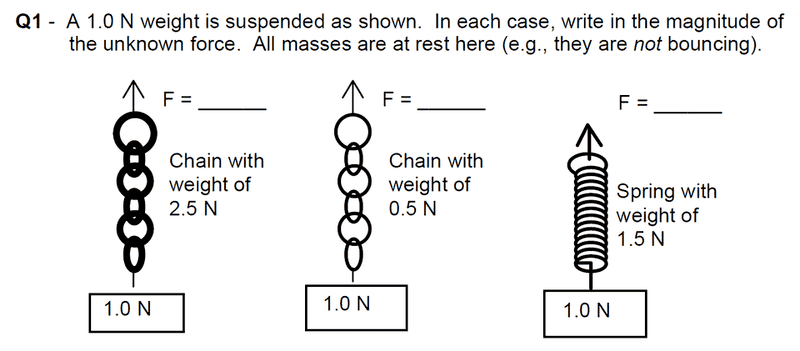# Simple Suspended Weight Force

• mohabitar

#### mohabitarWe usually only dealt with blocks suspended from a weightless string, so this is new to me. What I did to find F for the first one, which I thing I did wrong is:
F[chain]=mg=2.5(9.8)=24.5
F[box]=1.0(9.8)=9.8
Then I added the two forces together. Is this correct, if not, where have I gone wrong?

I believe you have it correct. The measurement point is insensitive to the location of the mass. Otherwise, you would need to consider such nonsense as the top half of each 1.0 N box versus its bottom half. Consider that your string is connected to the top of the heavy chain, light chain or spring. In each case, the 'string' remains weightless.

No offense at all, I just want to make sure for sure that its right, but can someone with more forum experience comment on this?

Your method is correct, all systems are in rest so the resultant force has to be zero. The only forces are gravity and the opposite force F. But you are given the weights (in Newtons) which are forces already: mass times g. Do not multiply them with g.

ehild

Wait so is my answer right?

No. The numerical results are wrong.

ehild

So weight=mg, so if they say the weight is 5N, they already made that calculation with gravity? So then I'd just add 2.5+1, or subtract? Which one and why?

Yes they have made the calculation with gravity. That is why the unity is N. If you multiply Newtons with m/s^2 you do not get force.

So the force of gravity are given for the chain and for the block, both point downward. You need the resultant force for the whole system: what is the resultant of two downward forces? Why do you want to subtract them?

ehild

ehild

Yes they have made the calculation with gravity. That is why the unity is N. If you multiply Newtons with m/s^2 you do not get force.

So the force of gravity are given for the chain and for the block, both point downward. You need the resultant force for the whole system: what is the resultant of two downward forces? Why do you want to subtract them?

ehild